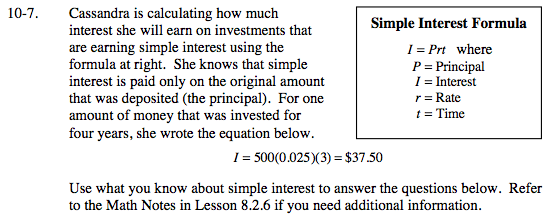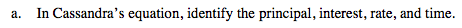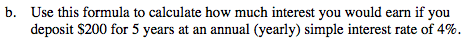### Home > MC2 > Chapter 10 > Lesson 10.1.1 > Problem10-7

10-7.Based on the simple interest formula given above, match the principal, interest, rate, and
time with the corresponding parts from Cassandra's equation.Substitute each part into the simple interest formula and solve.

I = (\$200)(.04)(5 years)